• Slides: 24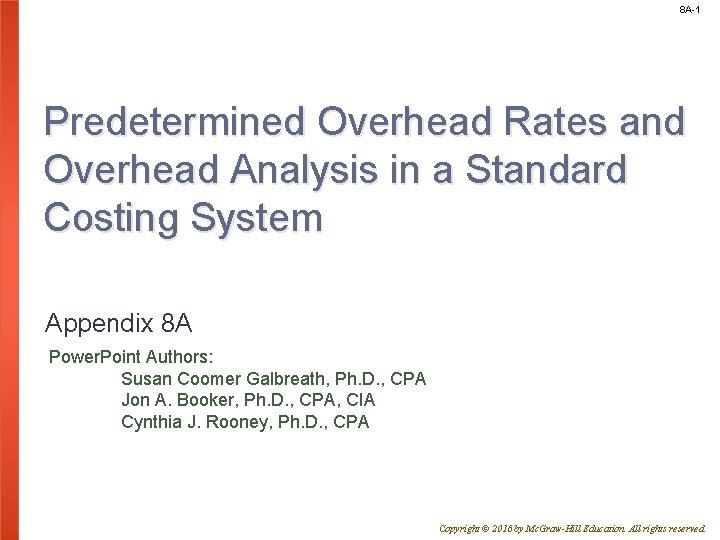8 A-1 Predetermined Overhead Rates and Overhead Analysis in a Standard Costing System Appendix 8 A Power. Point Authors: Susan Coomer Galbreath, Ph. D. , CPA Jon A. Booker, Ph. D. , CPA, CIA Cynthia J. Rooney, Ph. D. , CPA Copyright © 2016 by Mc. Graw-Hill Education. All rights reserved.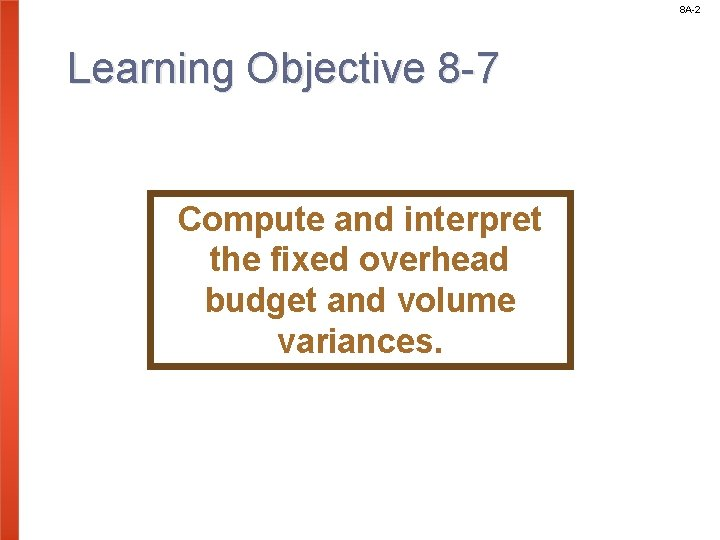8 A-2 Learning Objective 8 -7 Compute and interpret the fixed overhead budget and volume variances.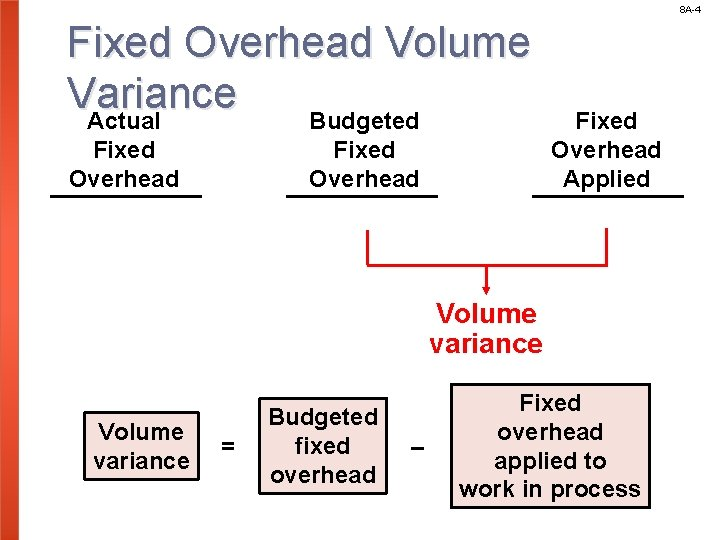8 A-4 Fixed Overhead Volume Variance Actual Budgeted Fixed Overhead Applied Volume variance = Budgeted fixed overhead – Fixed overhead applied to work in process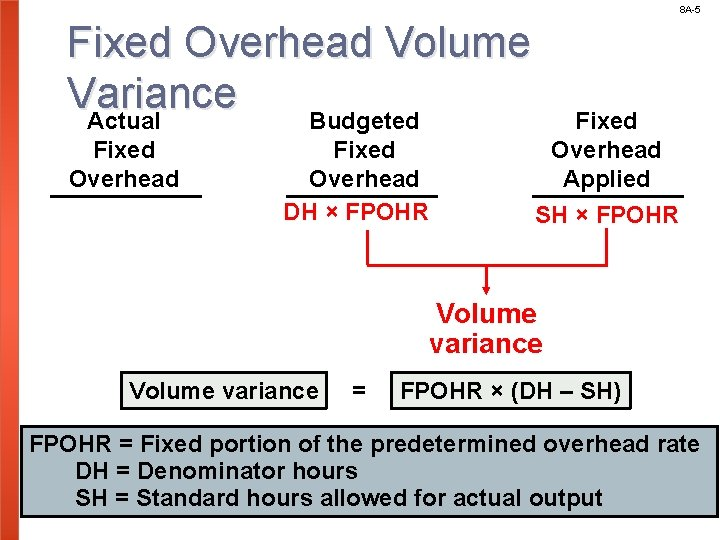8 A-5 Fixed Overhead Volume Variance Actual Budgeted Fixed Overhead DH × FPOHR Fixed Overhead Applied SH × FPOHR Volume variance = FPOHR × (DH – SH) FPOHR = Fixed portion of the predetermined overhead rate DH = Denominator hours SH = Standard hours allowed for actual output8 A-6 Computing Fixed Overhead Variances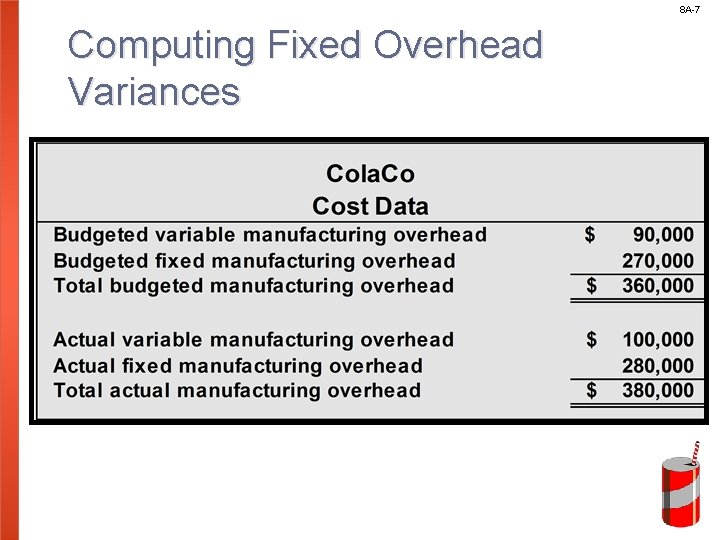8 A-7 Computing Fixed Overhead Variances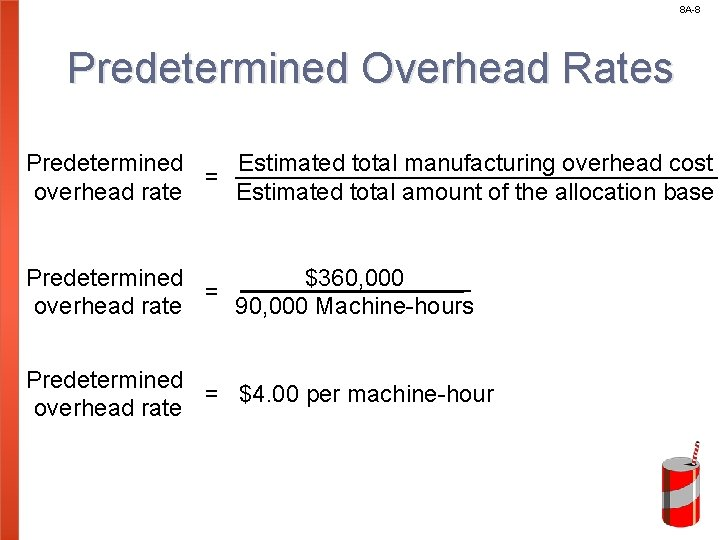8 A-8 Predetermined Overhead Rates Predetermined Estimated total manufacturing overhead cost = overhead rate Estimated total amount of the allocation base \$360, 000 Predetermined = 90, 000 Machine-hours overhead rate Predetermined = \$4. 00 per machine-hour overhead rate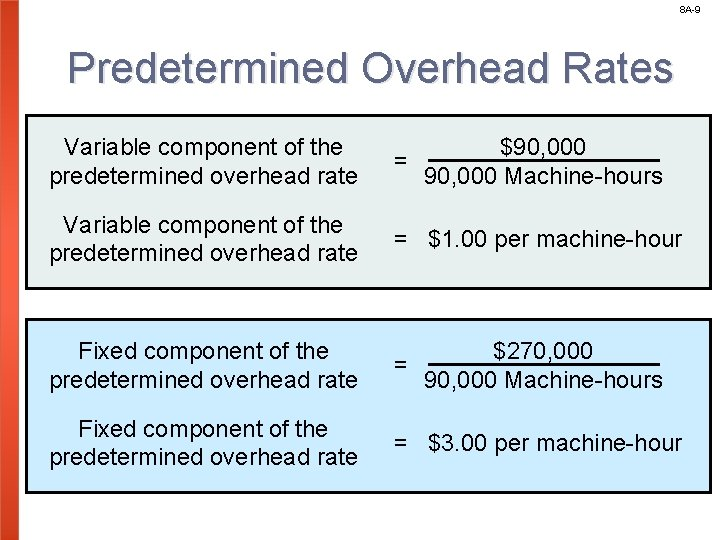8 A-9 Predetermined Overhead Rates Variable component of the predetermined overhead rate \$90, 000 = 90, 000 Machine-hours Variable component of the predetermined overhead rate = \$1. 00 per machine-hour Fixed component of the predetermined overhead rate \$270, 000 = 90, 000 Machine-hours Fixed component of the predetermined overhead rate = \$3. 00 per machine-hour8 A-10 Applying Manufacturing Overhead applied = Predetermined overhead rate × Standard hours allowed for the actual output Overhead applied = \$4. 00 per machine-hour × 84, 000 machine-hours Overhead applied = \$336, 0008 A-11 Computing the Budget Variance Actual fixed overhead Budgeted fixed overhead Budget variance = \$280, 000 – \$270, 000 Budget variance = \$10, 000 Unfavorable –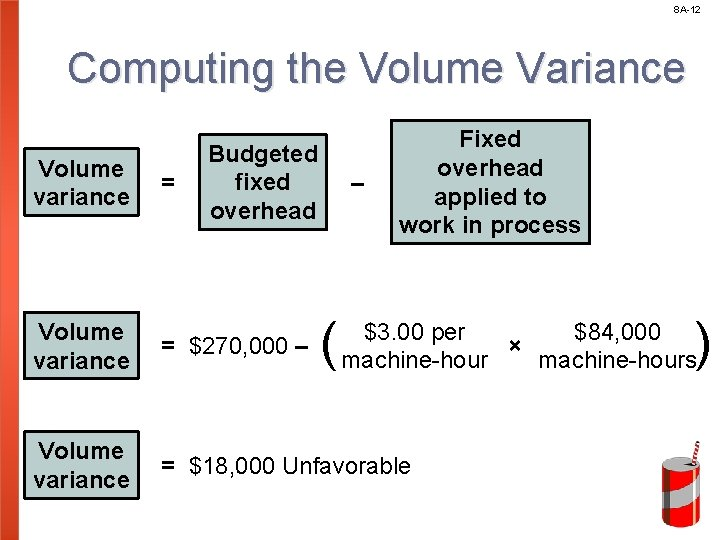8 A-12 Computing the Volume Variance Volume variance = Budgeted fixed overhead – ( Fixed overhead applied to work in process ) \$3. 00 per \$84, 000 × machine-hours Volume variance = \$270, 000 – Volume variance = \$18, 000 Unfavorable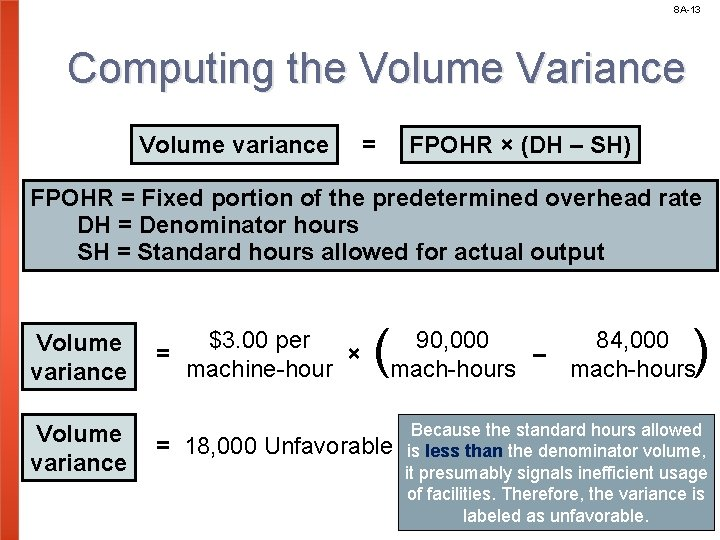8 A-13 Computing the Volume Variance Volume variance = FPOHR × (DH – SH) FPOHR = Fixed portion of the predetermined overhead rate DH = Denominator hours SH = Standard hours allowed for actual output Volume variance \$3. 00 per = × machine-hour ( ) 90, 000 84, 000 – mach-hours = 18, 000 Unfavorable Because the standard hours allowed is less than the denominator volume, it presumably signals inefficient usage of facilities. Therefore, the variance is labeled as unfavorable.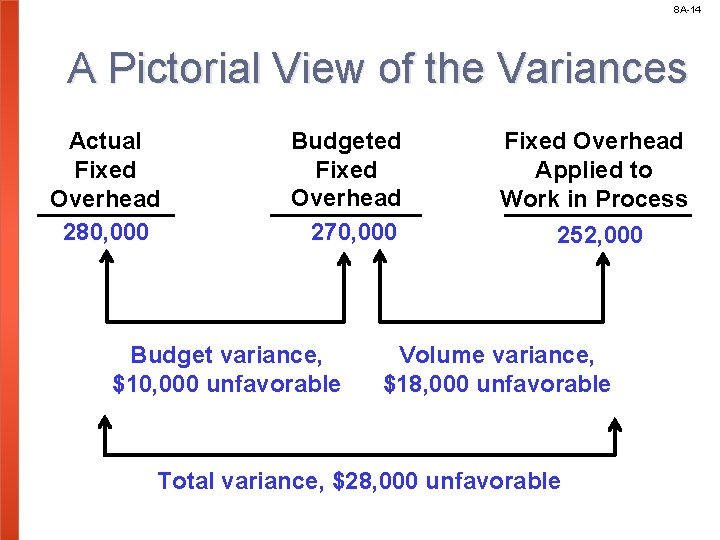8 A-14 A Pictorial View of the Variances Actual Fixed Overhead 280, 000 Budgeted Fixed Overhead 270, 000 Budget variance, \$10, 000 unfavorable Fixed Overhead Applied to Work in Process 252, 000 Volume variance, \$18, 000 unfavorable Total variance, \$28, 000 unfavorable8 A-15 Fixed Overhead Variances – A Graphic Approach Let’s look at a graph showing fixed overhead variances. We will use Cola. Co’s numbers from the previous example.8 A-16 Graphic Analysis of Fixed Overhead Variances Budget \$270, 000 t a ed i l r p u p o a h d d a r e a h er tand v o s r d e e x p i F 0 0. \$3 Denominator hours 0 0 Machine-hours (000) 908 A-17 Graphic Analysis of Fixed Overhead Variances Actual \$280, 000 Budget \$270, 000 { Budget Variance 10, 000 U t a ed i l r p u p o a h d d a r e a h er tand v o s r d e e x p i F 0 0. \$3 Denominator hours 0 0 Machine-hours (000) 908 A-18 Graphic Analysis of Fixed Overhead Variances Actual \$280, 000 Budget \$270, 000 Applied \$252, 000 { { Budget Variance 10, 000 U Volume Variance 18, 000 U t a ed i l r p u p o a h d d a r e a h er tand v o s r d e e x p i Standard F 0. 0 hours 3 \$ Denominator hours 0 0 Machine-hours (000) 84 908 A-19 Reconciling Overhead Variances and Underapplied or Overapplied Overhead In a standard cost system: Unfavorable variances are equivalent to underapplied overhead. Favorable variances are equivalent to overapplied overhead. The sum of the overhead variances equals the under- or overapplied overhead cost for the period.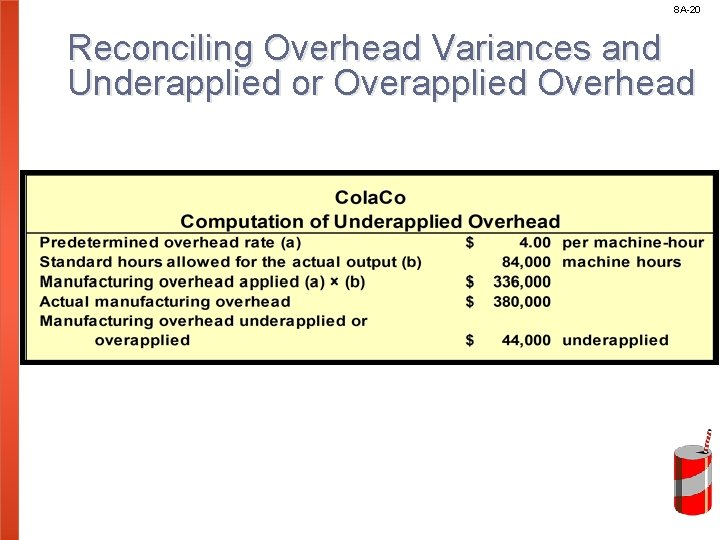8 A-21 Computing the Variable Overhead Variances Variable manufacturing overhead rate variance VMRV = (AH × AR) – (AH × SR) = \$100, 000 – (88, 000 hours × \$1. 00 per hour) = \$12, 000 unfavorable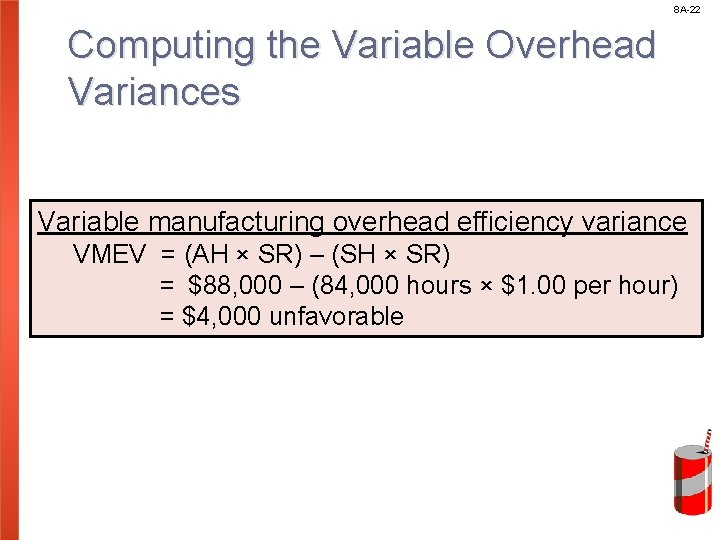8 A-22 Computing the Variable Overhead Variances Variable manufacturing overhead efficiency variance VMEV = (AH × SR) – (SH × SR) = \$88, 000 – (84, 000 hours × \$1. 00 per hour) = \$4, 000 unfavorable8 A-23 Computing the Sum of All Variances8 A-24 End of Chapter 8 A